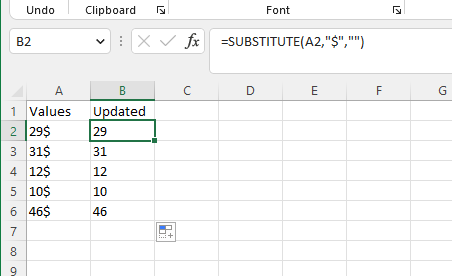# How to remove last char from a range in one go without loops

Hello Friends,

I want to remove “\$” sign in one go without any loops.
I tried below formula in write cell activity but showing error:-
“=SUBSTITUTE(C2,”\$“,”“)”

It shows wrong format.

Can we have any other approaches?

Can you please check below screenshot its working for me,

Use this below code in write cell activity,
`"=SUBSTITUTE(A2,""\$"","""")"`Hope this may help you5 Likes

Thanks @Manish540

Issue resolved.

If you found my answer useful to you please mark it as solution. I guess you marked wrong reply as solution.

Thank youOne more thing I want to ask.

Assume if we are getting this data from any Datatable not in excel so, If we want to remove \$ sign and want in int format hence we can do the sum. How we can do that?

Use this below code to sum the column values,
`sumOfValues(Variable type is Double) = dt.AsEnumerable().Sum(Function(x) CDbl(x("Values").ToString.Trim.Replace("\$","")))`

`sumOfValues(Variable type is Integer) = dt.AsEnumerable().Sum(Function(x) CInt(x("Values").ToString.Trim.Replace("\$","")))`

Hope this helpsYou are awesome bro,

This is what I am looking for.